5/26/2015

## Review: Comparing Variables

In previous chapters, we looked for relationships (associations) between variables by:

• Comparing categorical variables with contingency tables and stacked barplots
• Comparing numeric variables across groups with side-by-side boxplots
• Looked at how variables change over time with timeplots

In this chapter, we will:

• Look for relationships between two numeric variables

## The Data

Recall the Motor Trend Cars data from previous chapters:

##                    mpg cyl disp  hp    wt  qsec vs     am
## Mazda RX4         21.0   6  160 110 2.620 16.46  V   auto
## Mazda RX4 Wag     21.0   6  160 110 2.875 17.02  V   auto
## Datsun 710        22.8   4  108  93 2.320 18.61  S   auto
## Hornet 4 Drive    21.4   6  258 110 3.215 19.44  S manual
## Hornet Sportabout 18.7   8  360 175 3.440 17.02  V manual
## Valiant           18.1   6  225 105 3.460 20.22  S manual

We might want to know:

• Is there a relationship between engine displacement (size) and horsepower?
• Is weight related to fuel efficiency?

## Overview

How to we find relationships between numeric (quantitative) variables?

• Visually: using scatterplots
• Numerically: using the correlation coefficient
• Usually, we do both
• In this course, we will only focus on linear relationships

## Scatterplots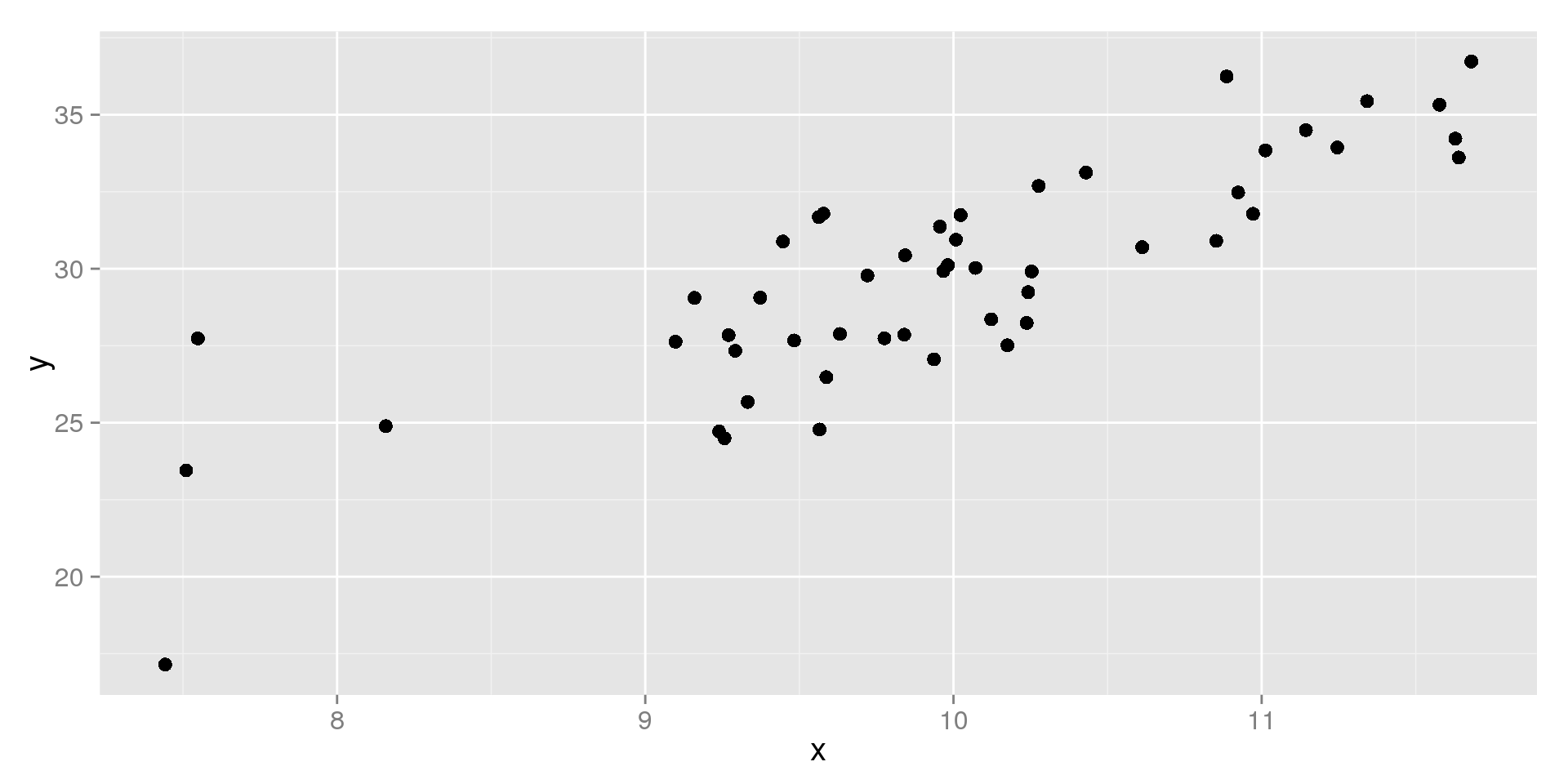## Scatterplots

How to make scatterplots:

• Define one variable as the $$X$$ variable, and one as $$Y$$
• Draw a point for each observation, using the values of the $$X$$ and $$Y$$ variables as coordinates
• Typically, the $$X$$ variable is on the horizontal axis and the $$Y$$ variable on the vertical axis

What we look for:

• Is there are trend or pattern?
• Are there any outliers or unusual points?

## Horsepower vs. Displacement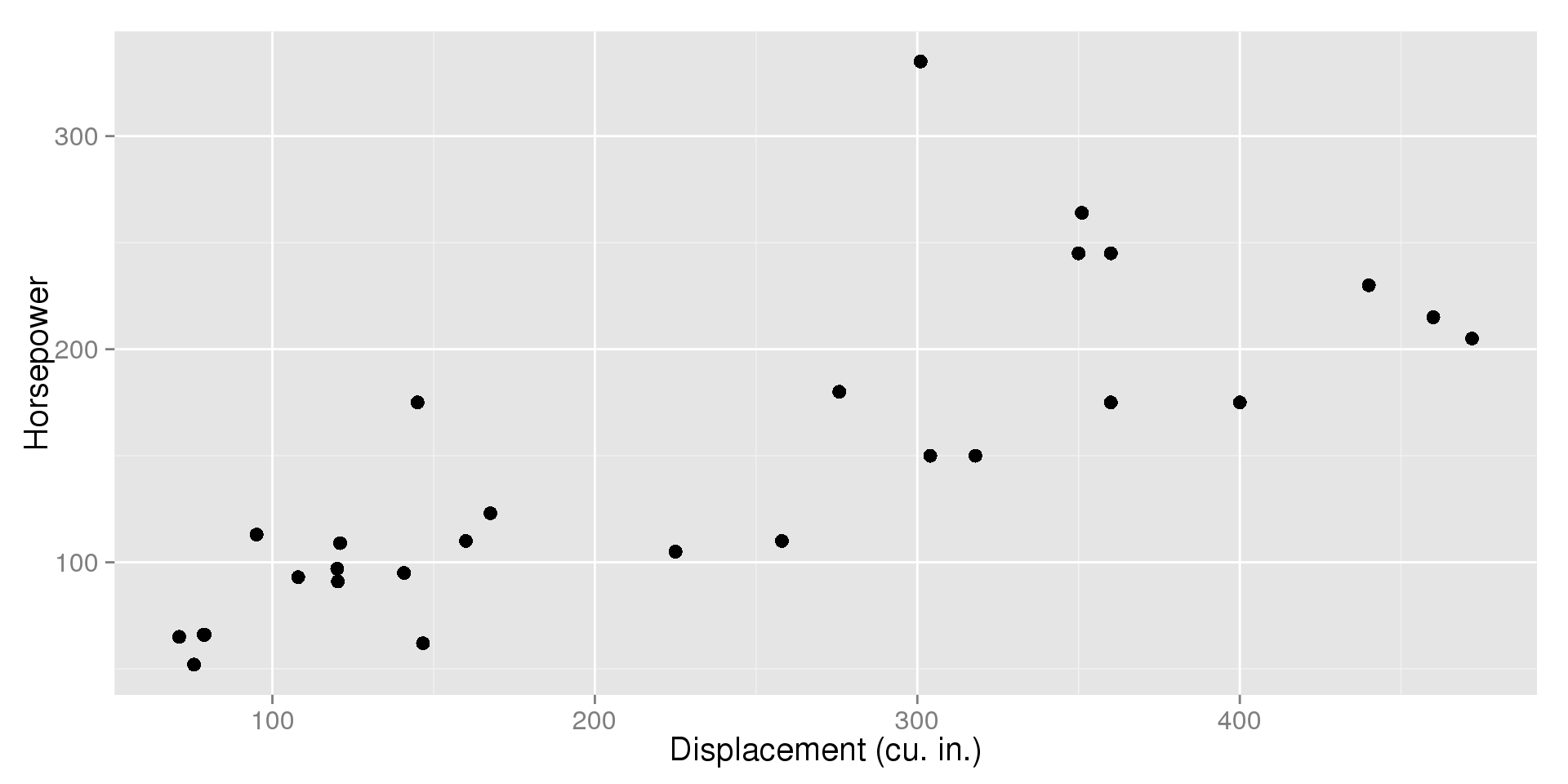## Horsepower vs. Displacement

Is there a relationship?

• As engines get bigger, they tend to have more horsepower
• We call this a positive association

Are there any unusual points?

• There is a point well above the rest
• Notice that it's engine size is right in the middle (about 300 cu. in.), but its horsepower is larger than any other car

## Weight vs. Fuel Efficiency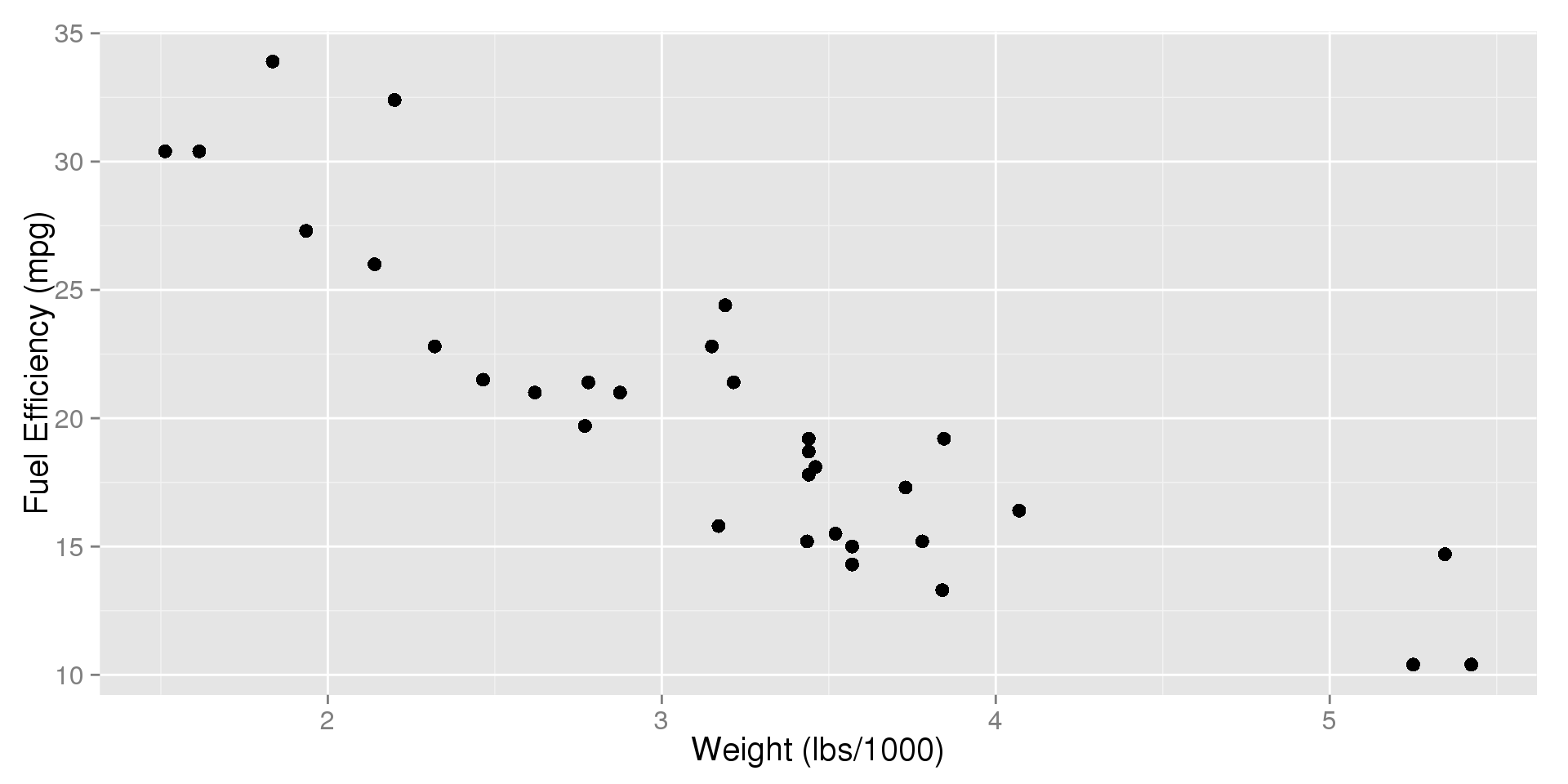## Weight vs. Fuel Efficiency

Is there a relationship?

• As cars get heavier, they tend to have lower fuel efficiency
• We call this a negative association

Are there any outliers?

• No points fall far away from the rest

## Types of Relationships

There are many types of trends that can come up when we make scatterplots. In this class, we will focus on the most common:

• Linear: The trend can be described fairly well by a straight line
• Non-linear: Any other type of trend

Directions of Relationships

• Positive: As one variable goes up, so does the other one
• Negative: As one variable goes up, the other goes down

Why lines?

• In statistics, we often try to find the simplest adequate method. Lines are simple.

## Strong Positive Linear Trend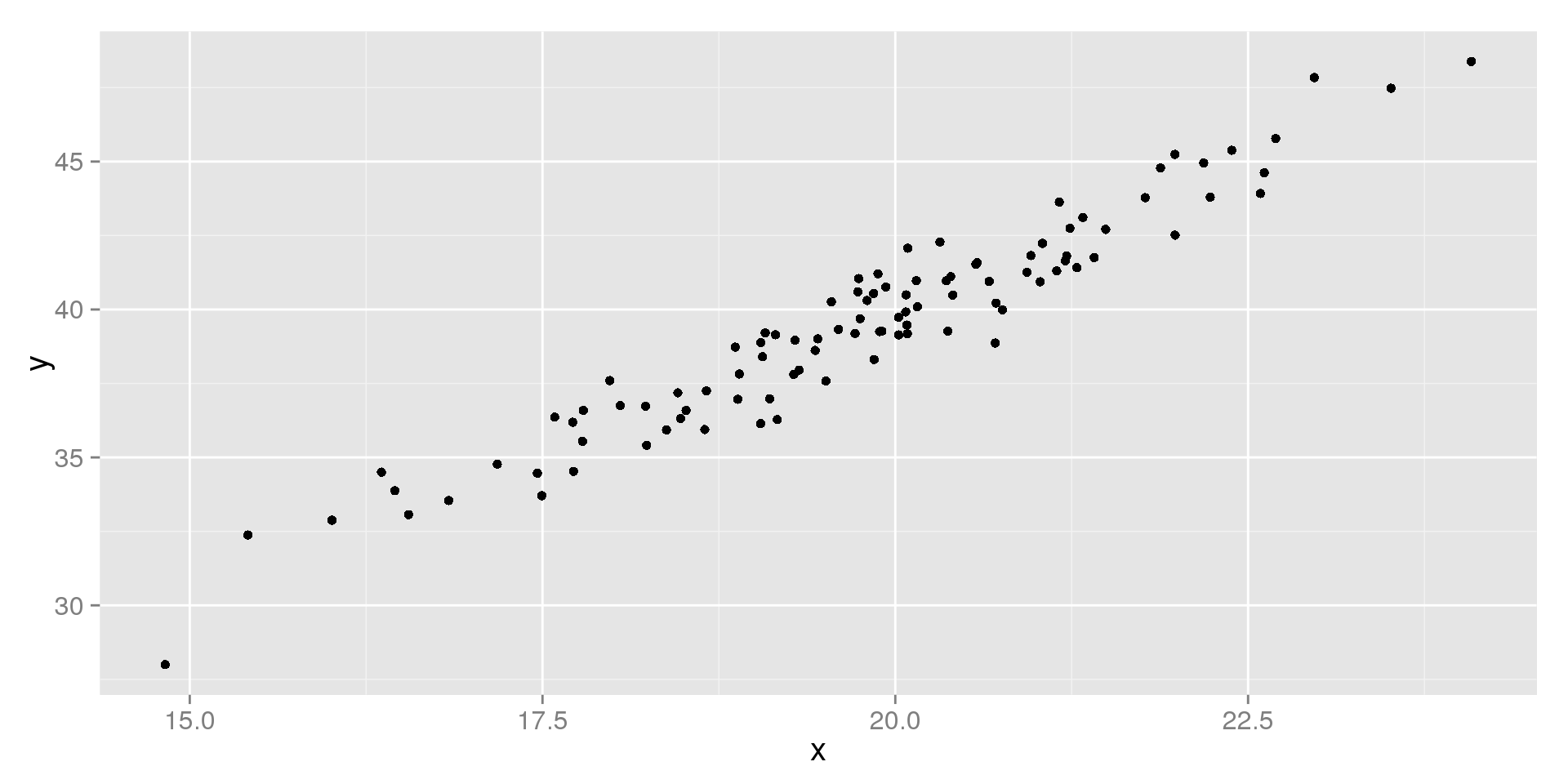## Moderate Negative Linear Trend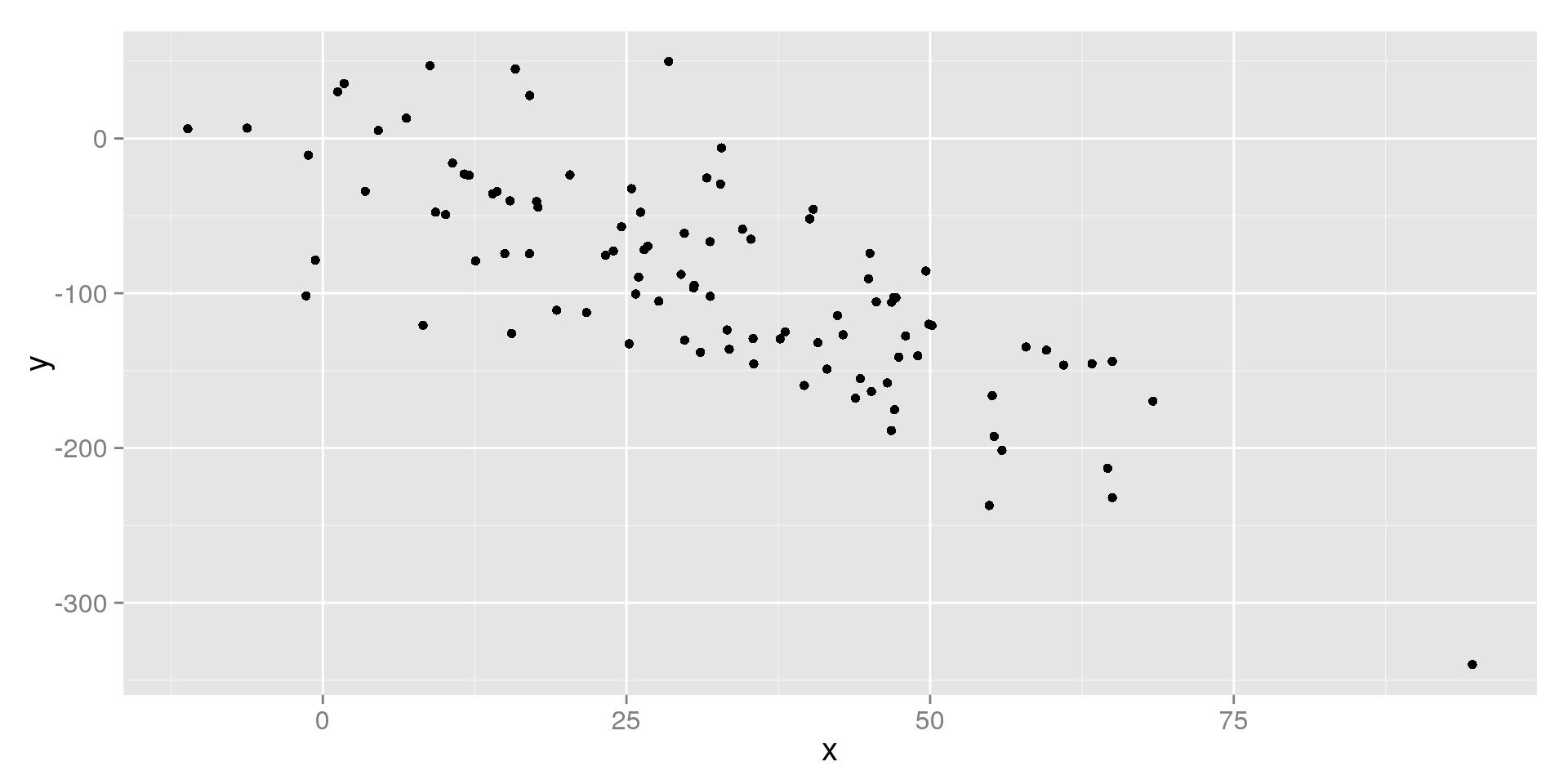## Weak Positive Linear Trend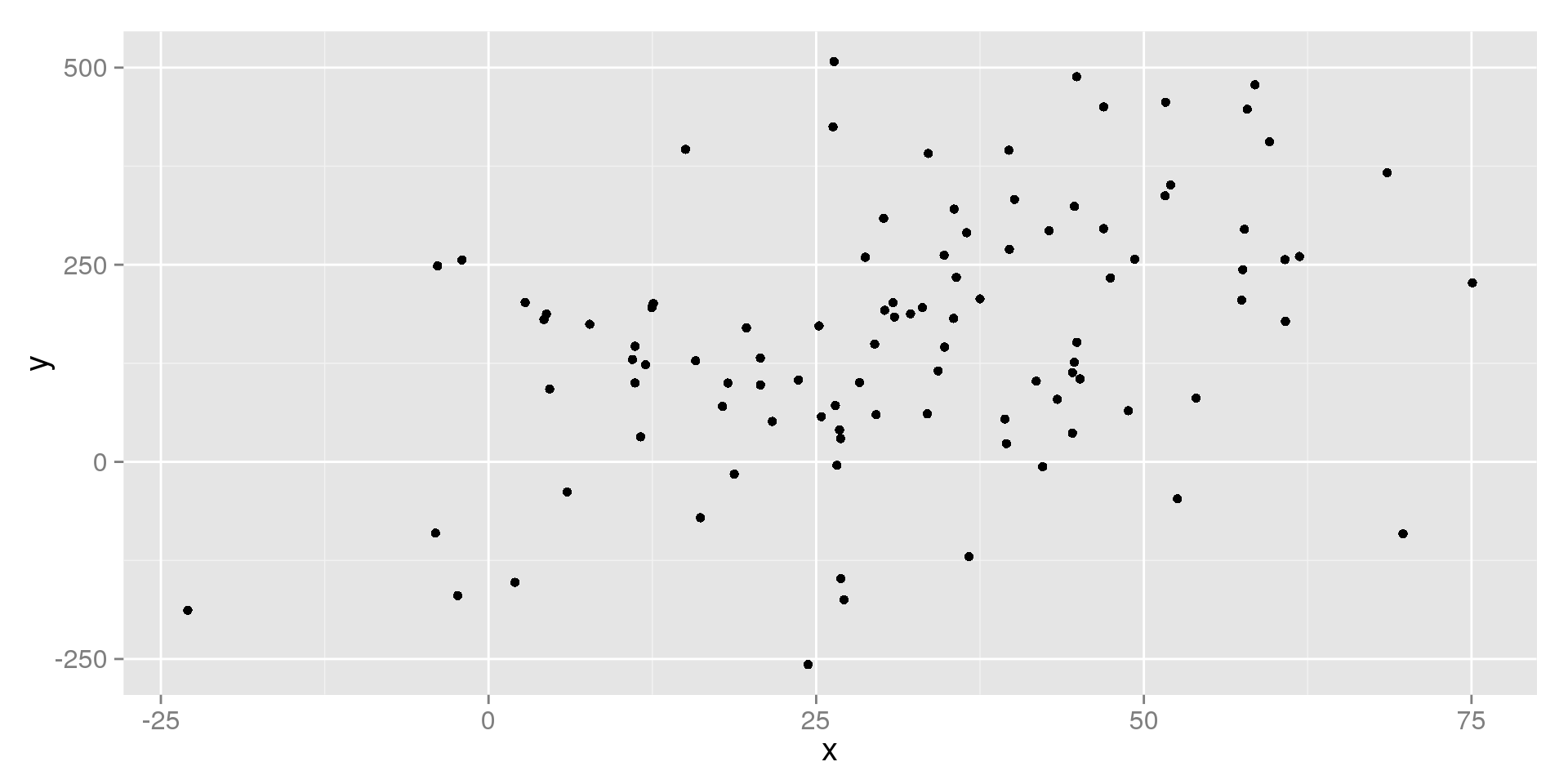## No Trend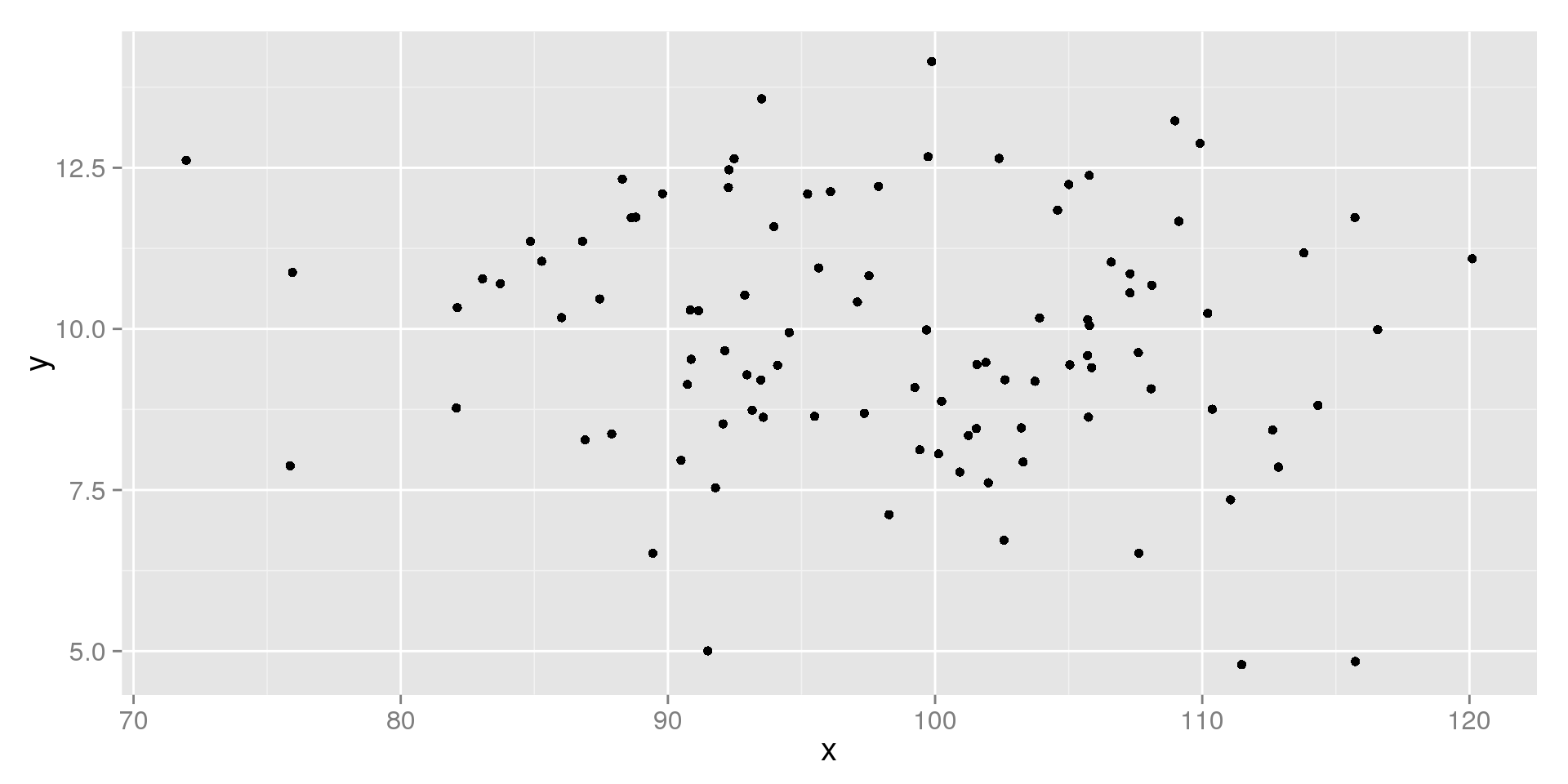## Non-Linear Trend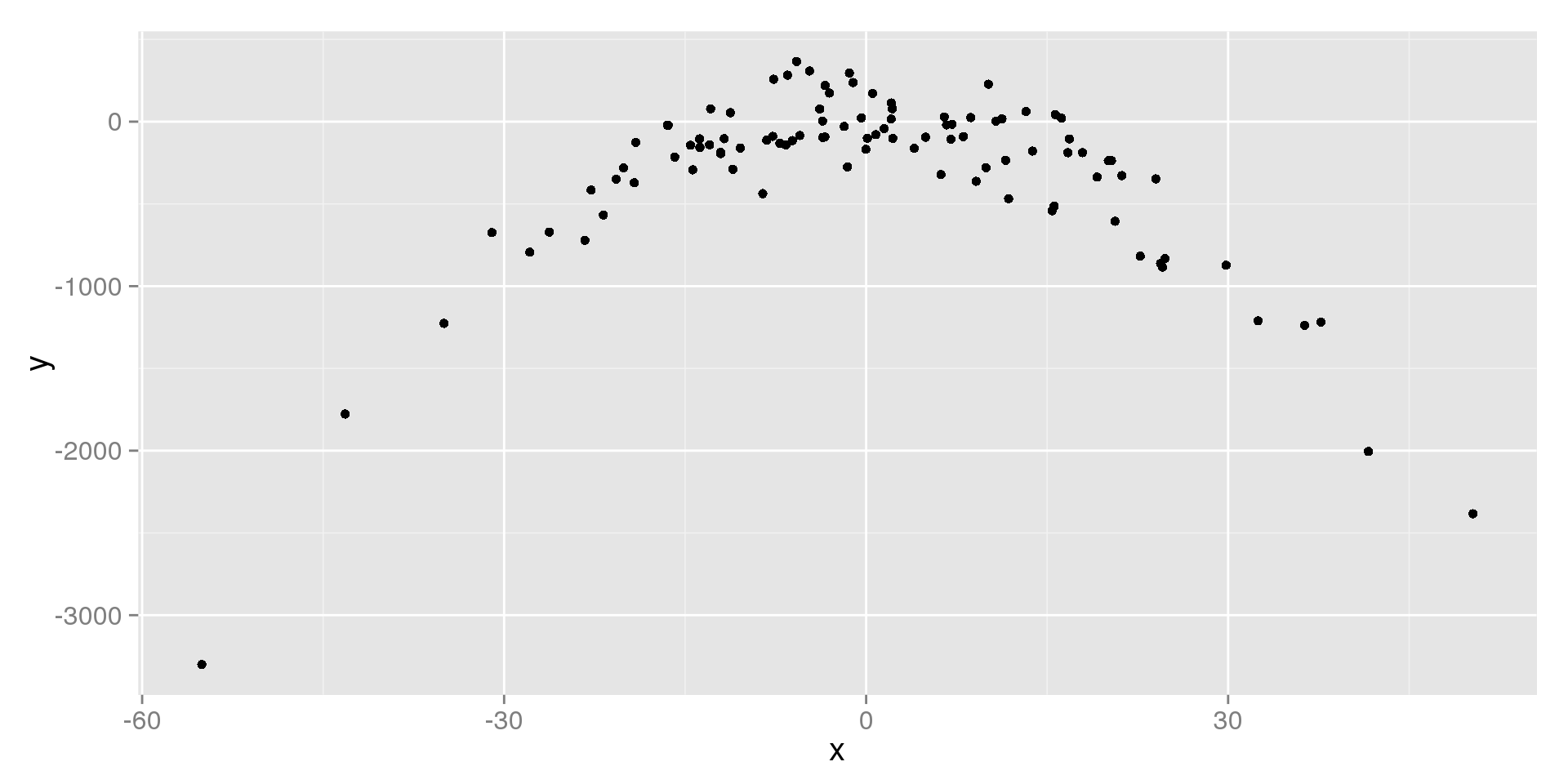## Non-Linear Trend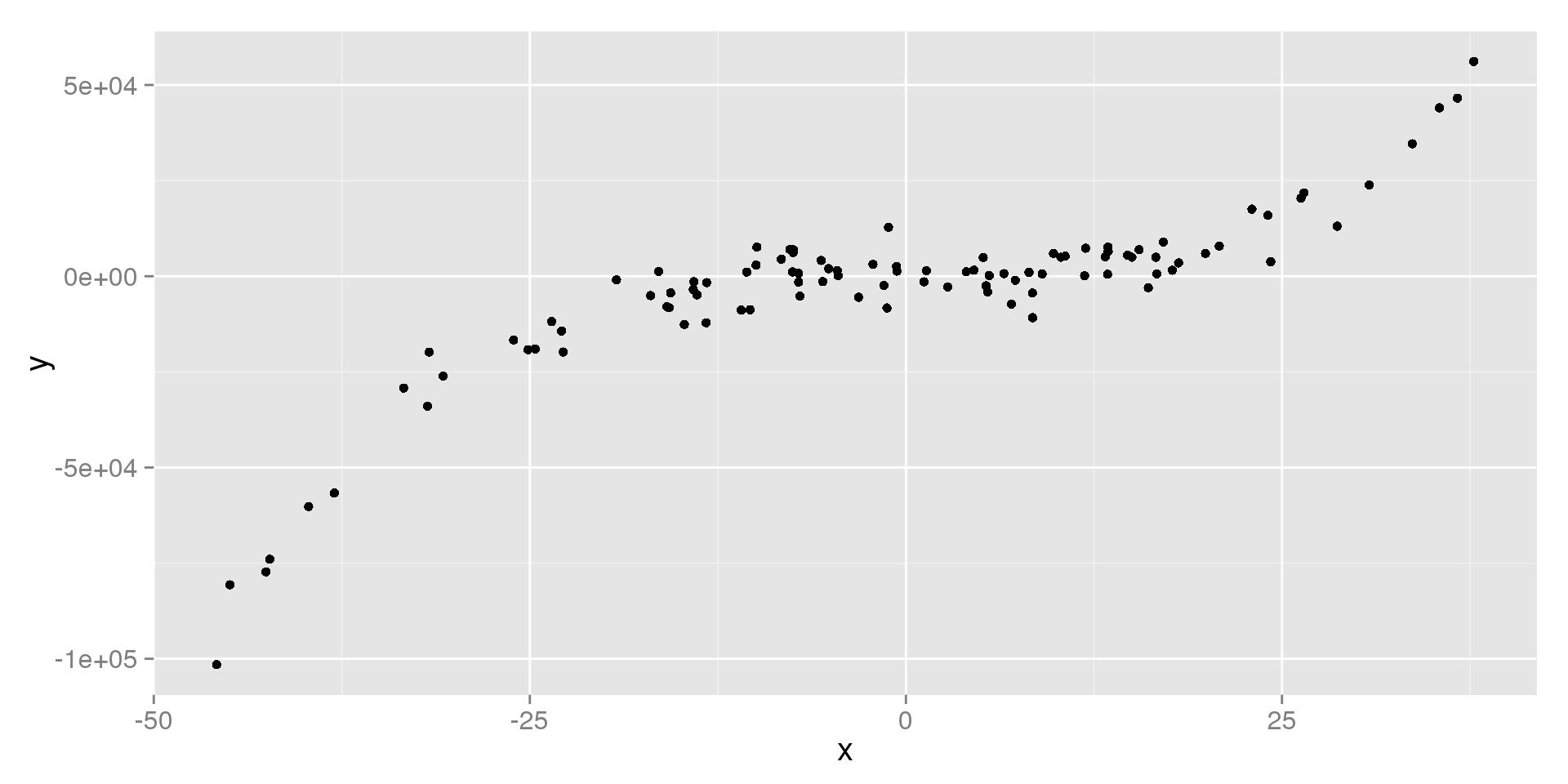## Roles of Variables

How do we decide which is $$X$$ and which is $$Y$$?

The $$X$$ Variable is:

• The explanatory or independent variable.
• We want to know if changes in this variable explains changes in $$Y$$

The $$Y$$ Variable is:

• The response or dependent variable
• We want to see if this variable responds when we change $$X$$

Which is which depends on what question we're asking.

## Variable Role Examples

Horsepower vs. Engine Displacement

• It makes sense that giving a car a bigger engine gives it more power.
• We can't just give a car more horsepower, horsepower responds to changes we make to the car.
• Horsepower should be $$Y$$, and Engine Displacement should be $$X$$.

Fuel Efficiency vs. Weight

• When we make a car heavier, it should mean that it takes more fuel to move it.
• Fuel efficiency responds to changes in the properties of the car.
• Fuel Efficiency should be $$Y$$, and Weight should be $$X$$.

## Measuring the Strength

How do we measure how strong the relationship is?

• We use the correlation coefficient
• $$r = \frac{\sum z_y \times z_x}{n-1}$$
• StatCrunch will find this for us

What is the correlation coefficient?

• $$r$$ is the strength of the linear relationship between two numeric variables
• It tells us how well a straight line explains the relationship

## Interpreting Correlation

• $$-1 \le r \le 1$$
• The value of $$r$$ tells us the strength
• The sign of $$r$$ tells us the direction
• $$r = 1$$: the points make a perfect straight line with a positive slope
• $$r = -1$$: the points make a perfect straight line with a negative slope
• $$r = 0$$: there is no linear relationship at all

Notes:

• You can sometimes get high correlations even if the relationship isn't linear
• You should always see a scatterplot along with a correlation coefficient to know whether or not it's meaningful

## Strong Positive Linear Trend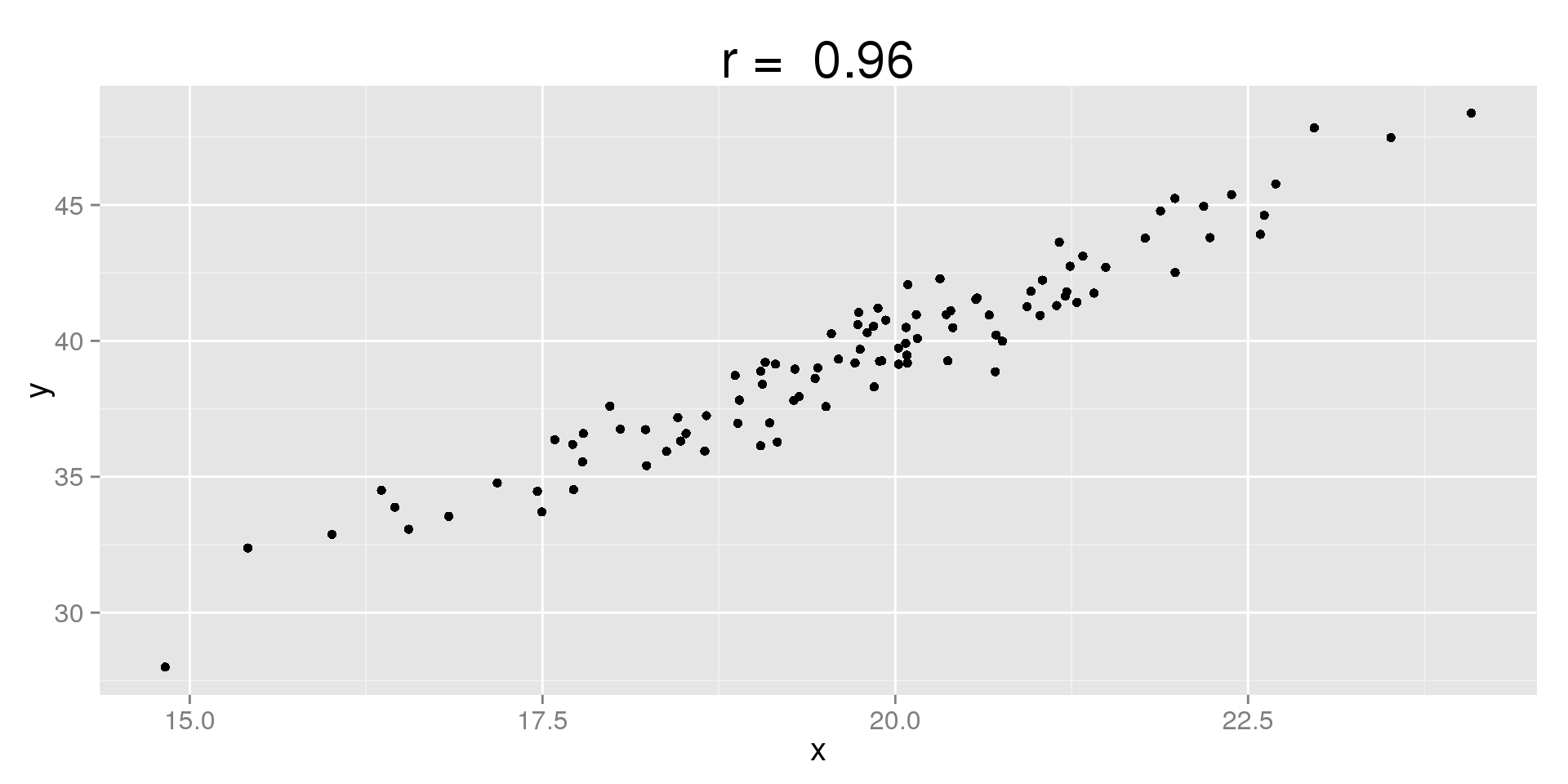## Moderate Negative Linear Trend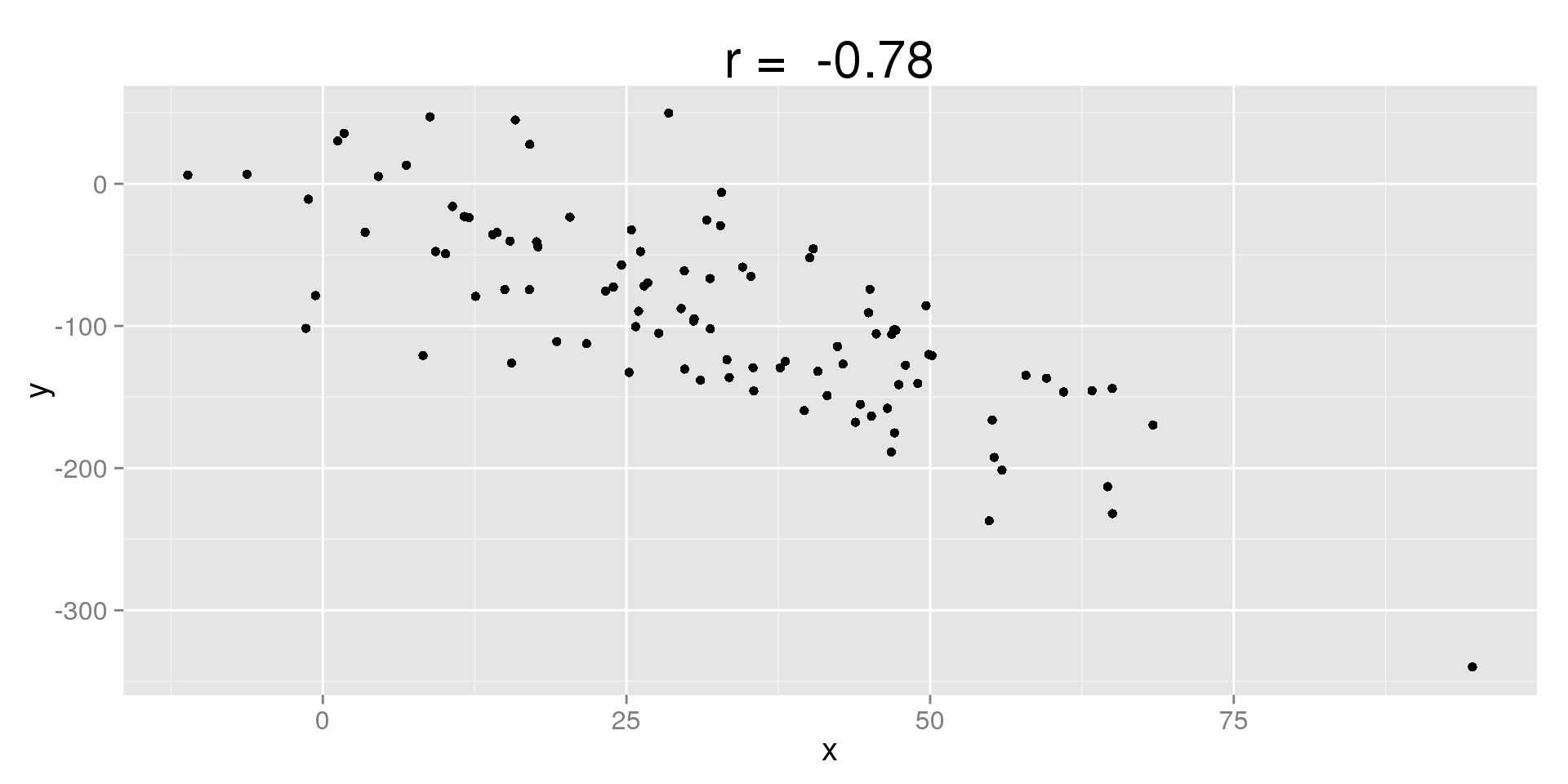## Weak Positive Linear Trend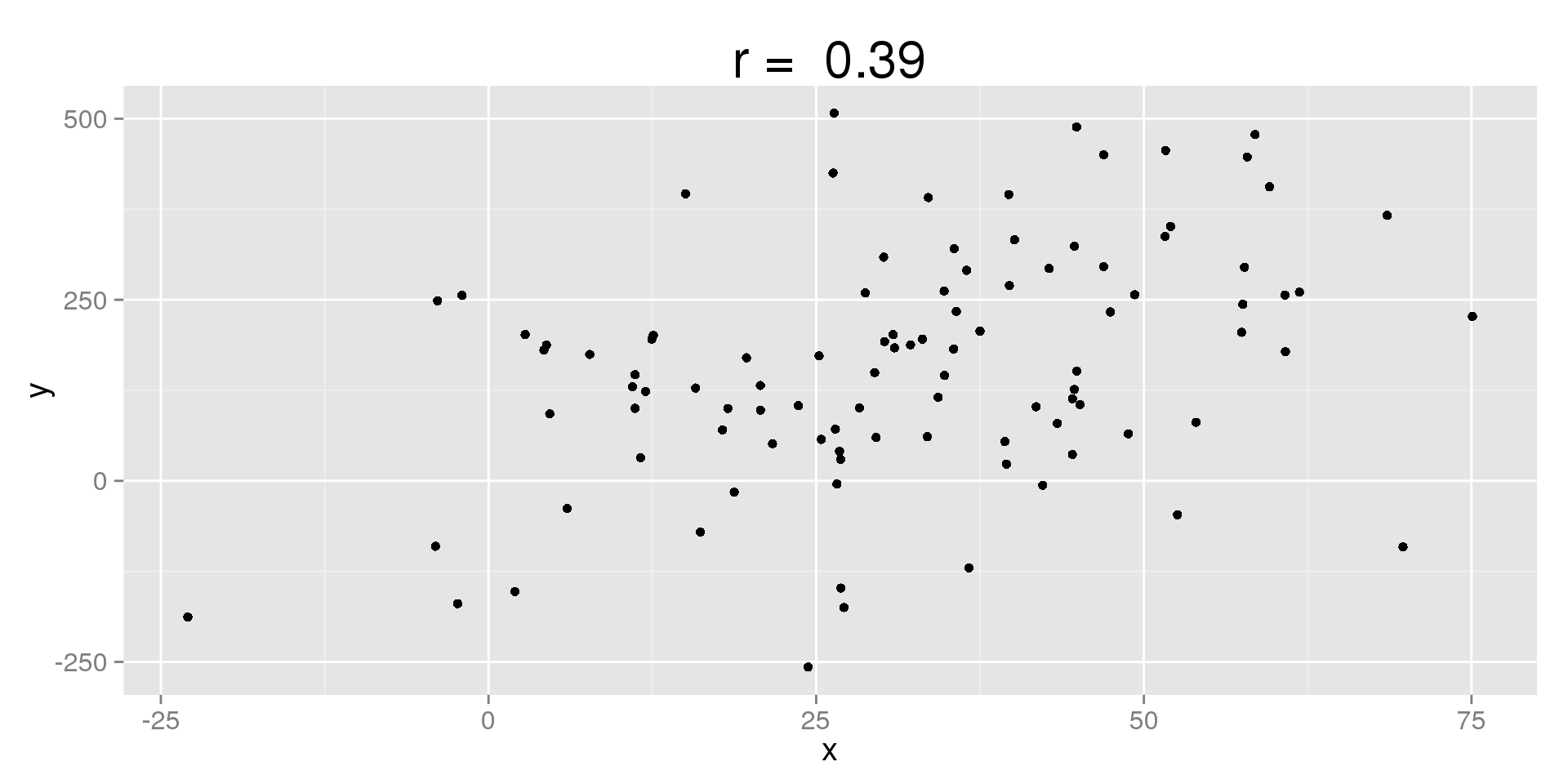## No Trend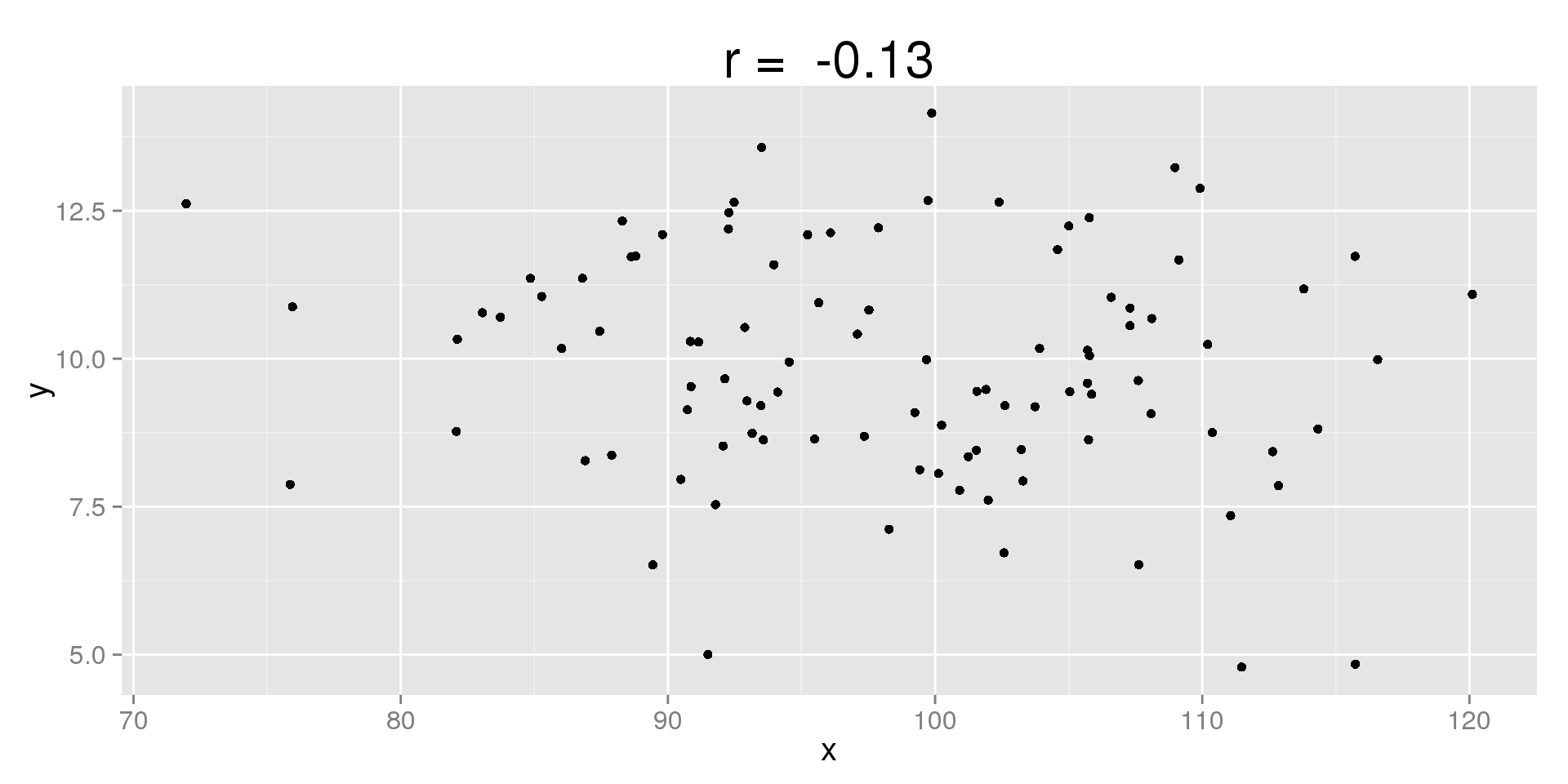## Non-Linear Trend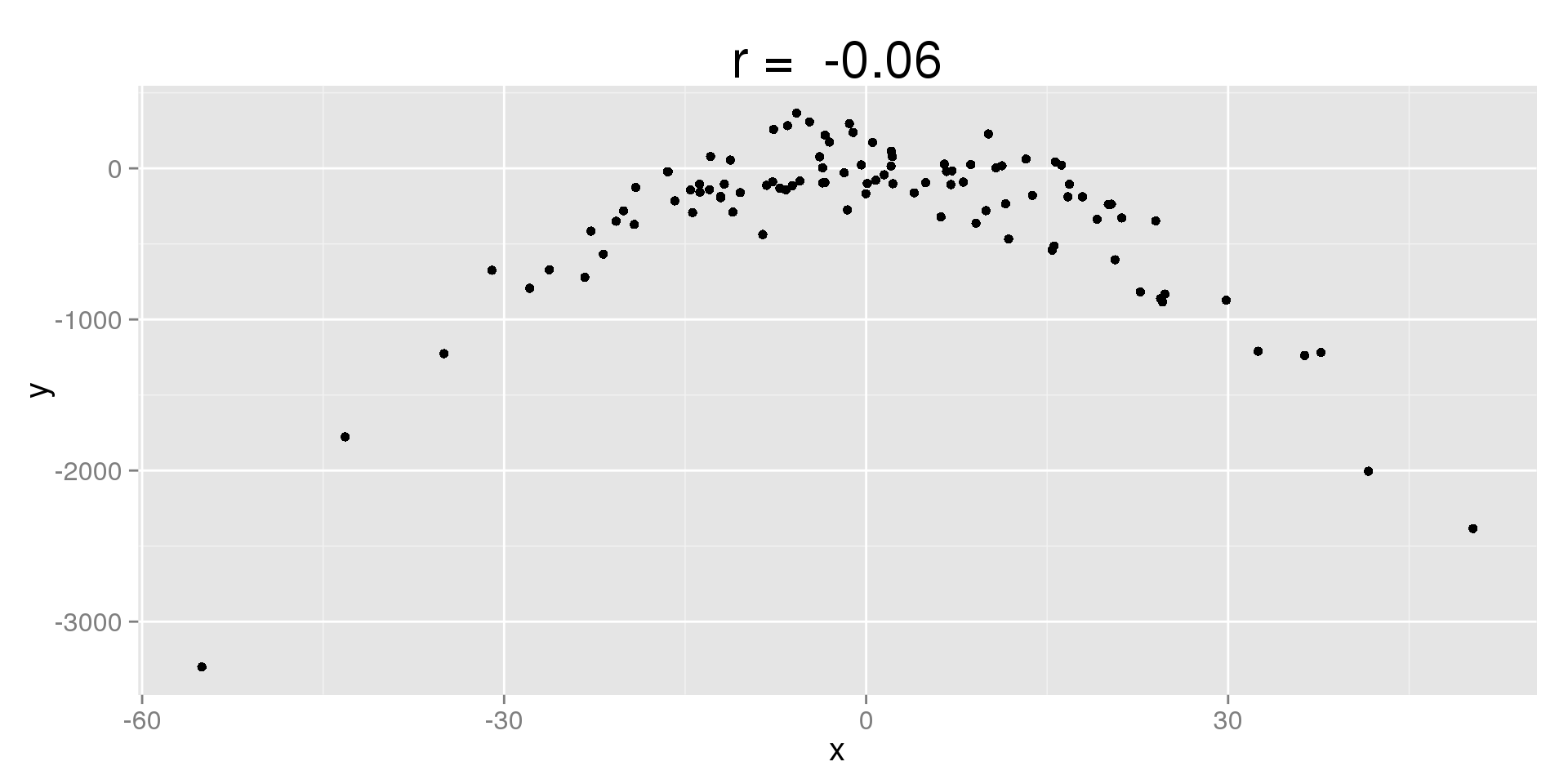## Non-Linear Trend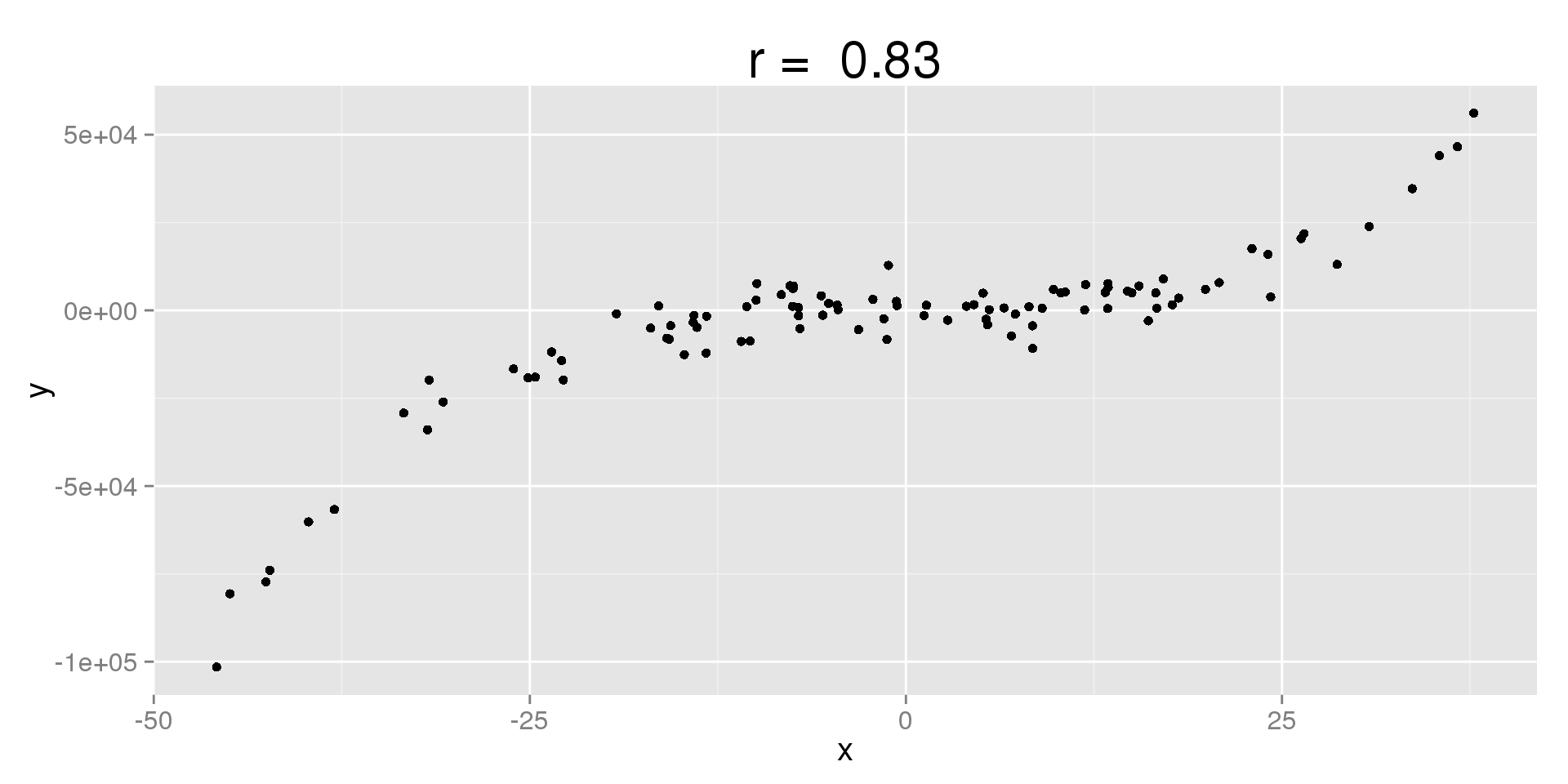## Using the Correlation Coefficient

So how do we use $$r$$?

• First, make a scatterplot
• There needs to be a linear association, or $$r$$ is meaningless
• Check the sign: is the relationship positive or negative?
• Check the value: how strong is the relationship?
• Are there outliers? The correlation is very sensitive to them.

Note:

• We often use the terms weak, moderate, and strong to describe the relationship, but these are up to interpretation.

## Outliers in Correlation

• $$r$$ is the strength of the overall linear relationship in the data
• If we have a point that is far away from the rest, it will decrease the strength of the relationship
• If we remove an outlier, it will drive $$r$$ away from 0 and towards -1 or 1
• If we add an outlier, it will drive $$r$$ towards 1

These relationships also hold if we alter a point's values (e.g., correct a typo in the data set)

• Moving a point towards the rest improves $$r$$
• Moving it away from the rest punishes $$r$$

## Horsepower vs. Engine Displacement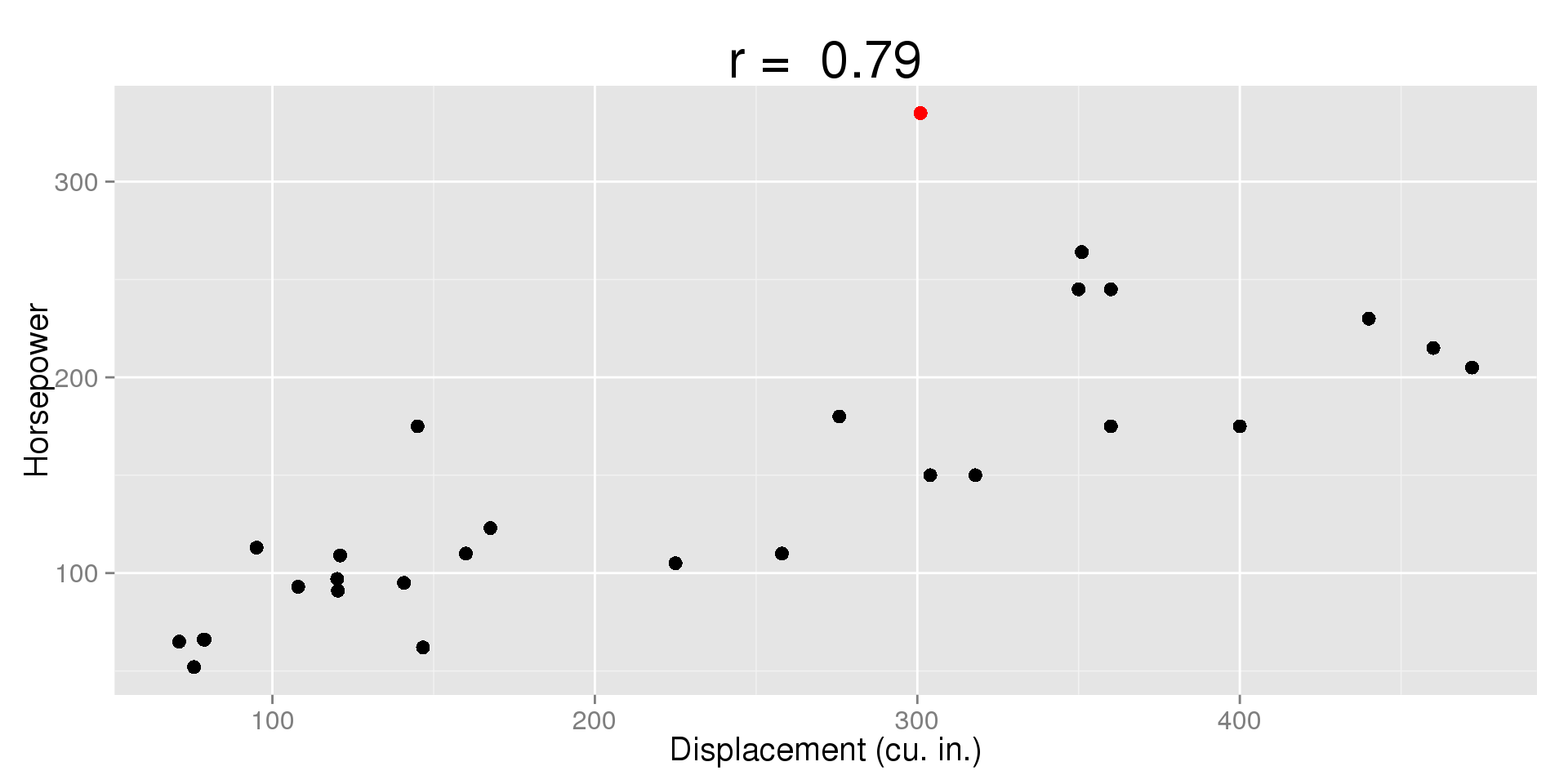## Horsepower vs. Engine Displacement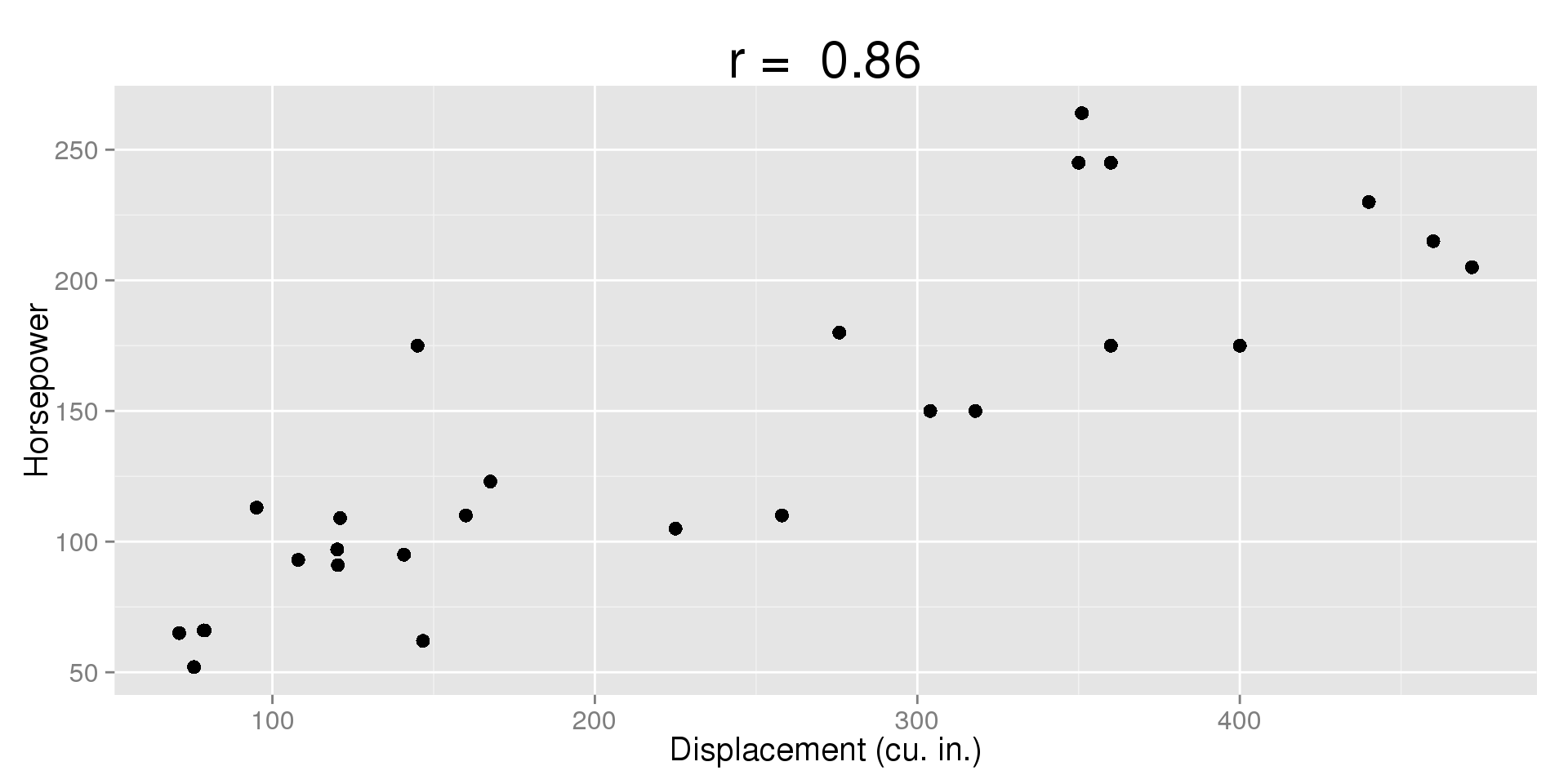## More Properties of Correlation

• $$r$$ is unitless
• $$r$$ is not affected by changes of center or scale
• If we change units, the correlation will not change (e.g., $$lbs \to kg$$)
• The correlation of $$X$$ and $$Y$$ is the same as the correlation between $$Z_x$$ and $$Z_y$$ (their z-scores)
• The correlation stays the same if we flip $$X$$ and $$Y$$
• Correlation only applies to relationships between numeric variables. If there is an association involving categorical variables, it is not correlation.

## Weight (lbs) vs. Fuel Efficiency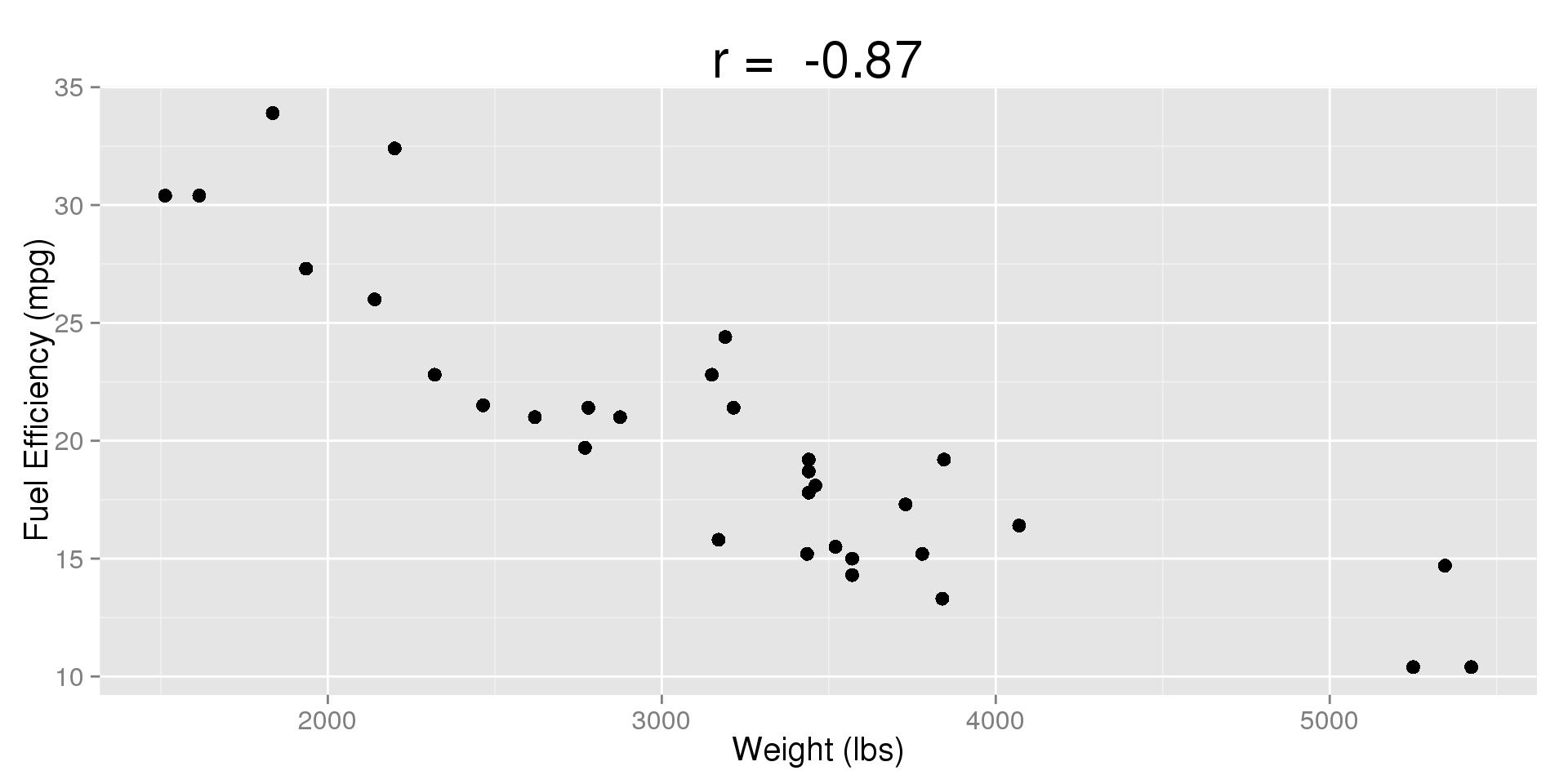## Weight (kg) vs. Fuel Efficiency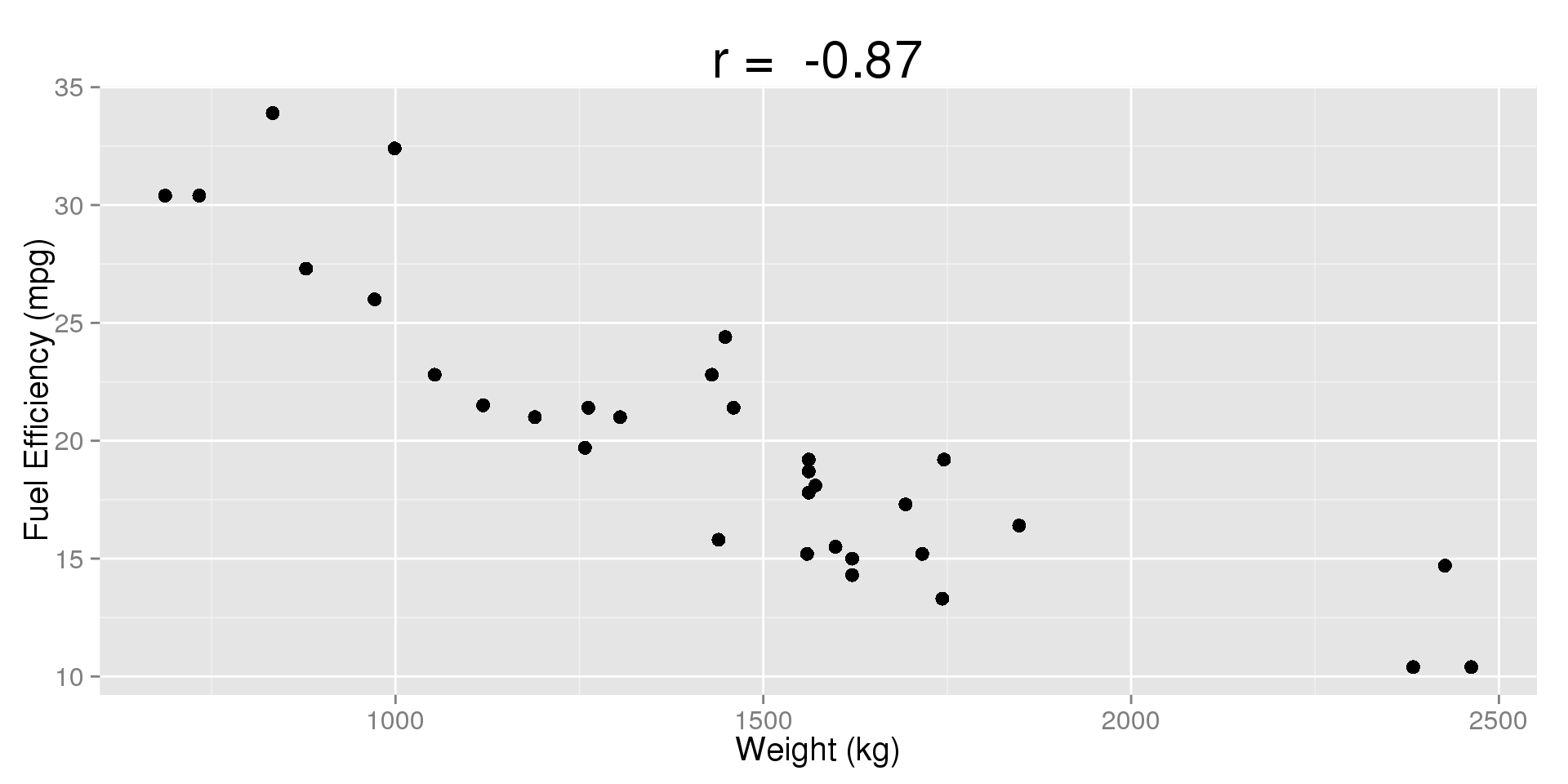## Weight vs. Fuel Efficiency (Z-Scores)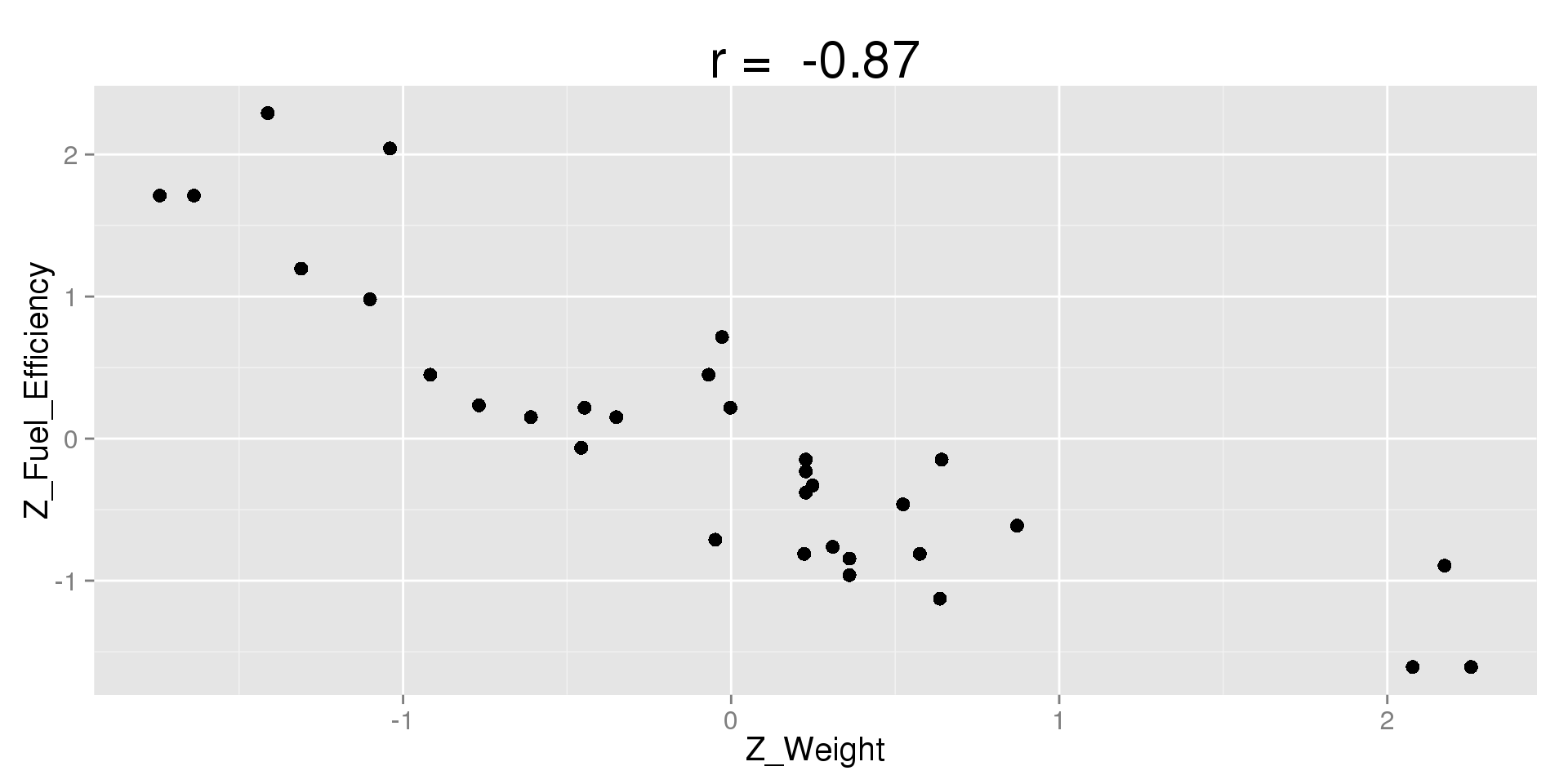## Fuel Efficiency vs. Weight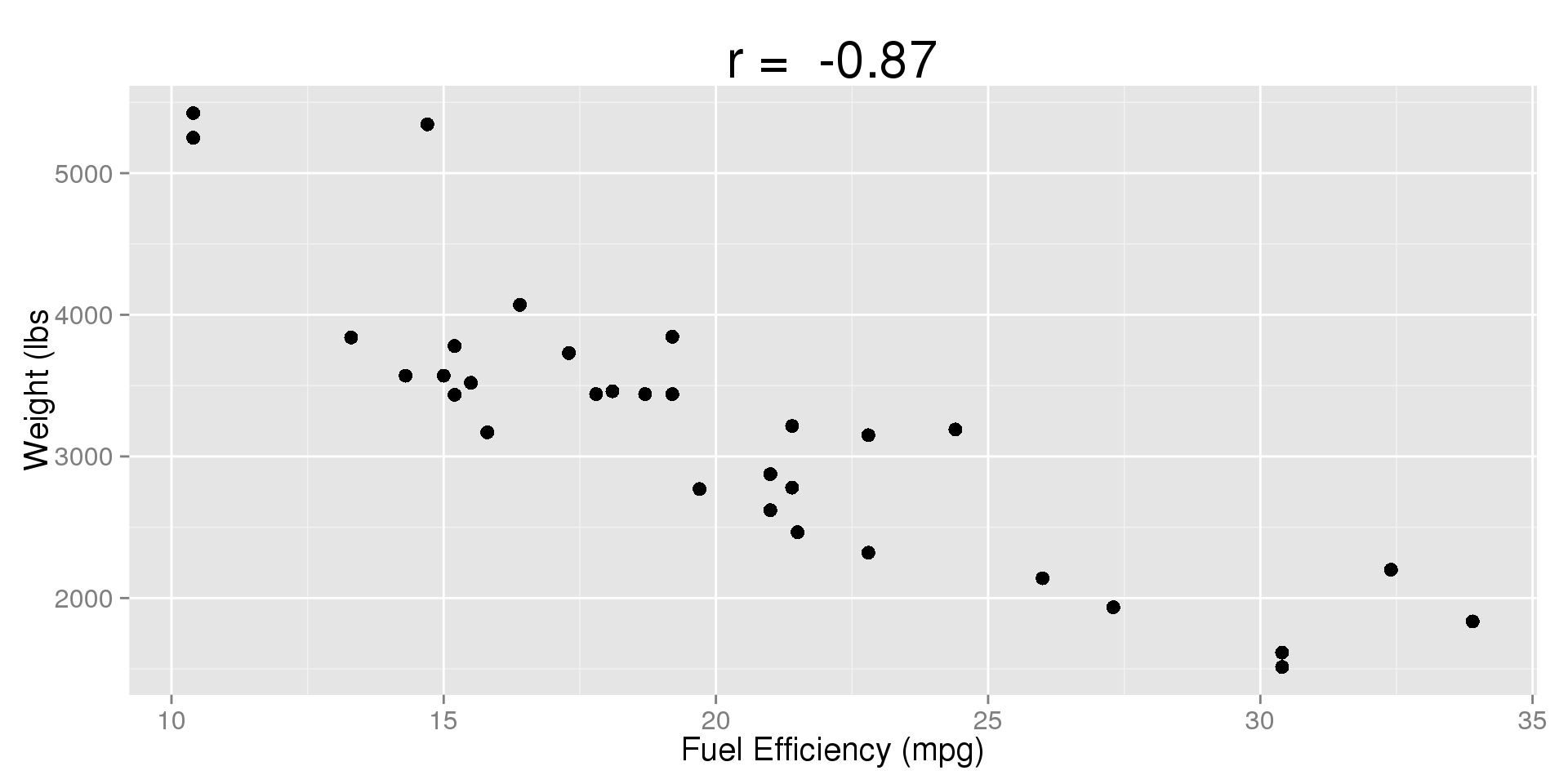## In StatCrunch

Scatterplots:

1. Graph $$\to$$ Scatter Plot
2. X Column $$\to$$ Select your explanatory $$(X)$$ variable
3. Y Column $$\to$$ Selected your response $$(Y)$$ variable
4. Compute!

Correlation:

1. Stat $$\to$$ Summary Stats $$\to$$ Correlation
2. Select Column(s) $$\to$$ Hold Shift/Ctrl/Command to select multiple variables (note: if you select more than two variables, it will find all pair-wise correlations)
3. Compute!

## Correlation $$\ne$$ Causation

Must people are familiar with the phrase "correlation does not equal causation," but what does that really mean?

• Even if we find a correlation between two variables, it does not mean that one causes the other.
• This is especially common when two things both increase or decrease over time.
• Both may be caused by other, unknown variables.
• We call these unknown variables lurking variables or confounding variables.

For example:

• What if we looked at the correlation between national ice cream sales and the number of forest fires, recorded for each month of the year?